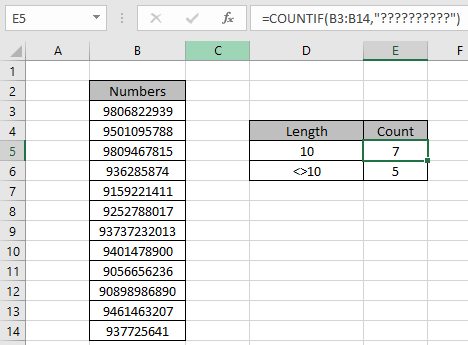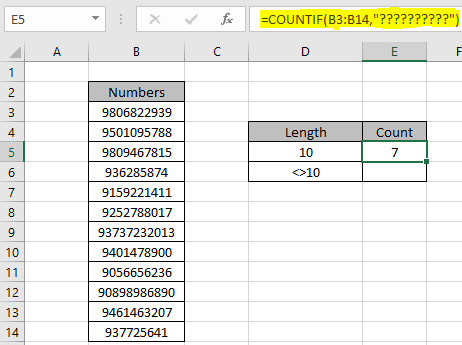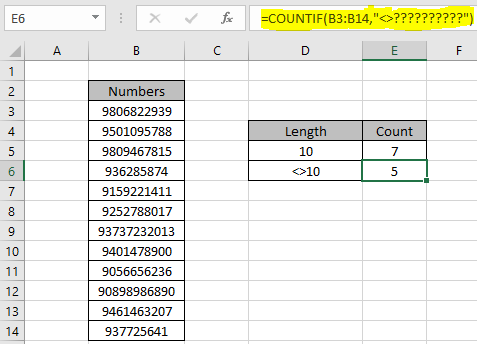# How to match number of characters in cell in ExcelIn this article, we will learn How to count cells which have a fixed number of string lengths in Excel.

Scenario :

At instances when working with datasets. Sometimes we need to just get how many cells in the list have exactly 5 characters. For Example finding the count of only 10 digit numbers in Excel or finding the count of numbers which doesn't have 10 digit numbers. Let's understand how we can solve this kind of problem using the COUNTIF function in Excel.

How to solve the problem?

For this, we will be using the COUNTIF function and wildcard ( ? ). COUNTIF function counts the number of matched cells which meet the criteria in the list. Here the criteria is the length of string be 10. String can have any datatype like date, time, names or numbers. (?) character matches a single uncertain character in string. Wildcards are used to match uncertain characters in Excel.

Generic formula:

 =COUNTIF(range,"?????")

range : list of values to match

????? : matches length of string equal to 5.

Example :

All of these might be confusing to understand. Let's understand this formula using it in an example. Here we have a list of numbers (12) having different lengths. We need to find the count of 10 digit numbers in Excel.

Use the formula:

 =COUNTIF(B3:B14,"??????????")There are 7 ten digit numbers in the list (B3:B14). As you can see in the formula stated there are 10 (?) characters which match exactly 10 digit numbers. For example matching time values which have hour value 9. So you can use "09:??.??". Each pair of question mark characters (?) are used for the uncertain minutes and seconds. You can also use the formula using the not equals to criteria like in the same example shown above if we need to find the number of cells which do not exactly have 10 characters in string.

Use the formula:

 =COUNTIF(B3:B14,"<>??????????")

<> : not equals to operator.As you can see there are 5 cells which do not have 10 digit numbers. You can also count the total rows using the count function then subtract the previous results to get the same result (12 - 7). If you have more than one criteria to match then use the COUNTIFS function in Excel.

Use the SUMPRODUCT function to count rows in the list. Learn more about Countif with SUMPRODUCT in Excel here.

Here are some observational notes shown below.

Notes:

1. The formula works with numbers, text, date values, etc.
2. Convert the list to text using =TEXT(value,"0") to count strings.
3. Use the same data type to match criteria in criteria list like name in names list, date in date values.
4. Lists as criteria ranges must be of same length, or the formula returns #VALUE! error.
5. Operators like equals to ( = ), less than equal to ( <= ), greater than ( > ) or not equals to ( <> ) can be used in criteria arguments, with numbers list only.
6. Use wildcards to match partial text matches in the list.

Hope this article about How to count cells which have a fixed number of string lengths in Excel is explanatory. Find more articles on Counting values in the table using formulas here. If you liked our blogs, share it with your friends on Facebook. And also you can follow us on Twitter and Facebook. We would love to hear from you, do let us know how we can improve, complement or innovate our work and make it better for you. Write us at info@exceltip.com

Related Articles :

How to use the SUMPRODUCT function in Excel: Returns the SUM after multiplication of values in multiple arrays in excel.

COUNTIFS with Dynamic Criteria Range : Count cells selecting the criteria from the list of options in criteria cell in Excel using data validation tool.

COUNTIFS Two Criteria Match : multiple criteria match in different lists in table using the COUNTIFS function in Excel

COUNTIFS With OR For Multiple Criteria : match two or more names in the same list using the OR criteria applied on the list in Excel.

How to Use Countif in VBA in Microsoft Excel : Count cells with criteria using Visual Basic for Applications code in Excel macros.

How to use wildcards in excel : Count cells matching phrases in text lists using the wildcards ( * , ? , ~ )in excel

Popular Articles:

How to use the IF Function in Excel : The IF statement in Excel checks the condition and returns a specific value if the condition is TRUE or returns another specific value if FALSE.

How to use the VLOOKUP Function in Exceln : This is one of the most used and popular functions of excel that is used to lookup value from different ranges and sheets.

How to use the COUNTIF Function in Excel : Count values with conditions using this amazing function. You don't need to filter your data to count specific values. Countif function is essential to prepare your dashboard.

How to use the SUMIF Function in Excel : This is another dashboard essential function. This helps you sum up values on specific conditions.

Terms and Conditions of use

The applications/code on this site are distributed as is and without warranties or liability. In no event shall the owner of the copyrights, or the authors of the applications/code be liable for any loss of profit, any problems or any damage resulting from the use or evaluation of the applications/code.